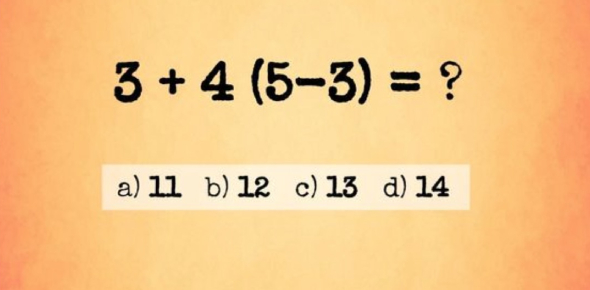# Maths Quiz For Class 2 With Answers

10 Questions | Total Attempts: 97372SettingsMath for some 2nd graders is the most challenging subject they have ever studied. The Maths quiz for class 2 with answers is designed to help you test out which problems to work more on. Give them a try and all the best as you continue to learn how to tackle more problems. Share your score.

• 1.
______ comes just before 413.
• A.

403

• B.

412

• C.

410

• D.

414

• 2.
In 289, the place value of 8 is____________.
• A.

Ones

• B.

Hundreds

• C.

Tens

• D.

Thousands

• 3.
Three hundred and one can be written as ______.
• A.

31

• B.

310

• C.

301

• D.

3001

• 4.
232, 242, ____ , 262. The missing number in the pattern is ____.
• A.

222

• B.

225

• C.

252

• D.

243

• 5.
10+43+34=
• A.

87

• B.

77

• C.

104334

• D.

197

• 6.
There are 3 sweets in one packet. How many sweets will be there in 9 packets?
• A.

12

• B.

27

• C.

9

• D.

3

• 7.
____ is 200 more than 546.
• A.

346

• B.

446

• C.

646

• D.

746

• 8.
There are 4 apples in each basket. How many apples will be there in 5 baskets?
• A.

5

• B.

20

• C.

9

• D.

10

• 9.
Jane bought 60 cherries. She put 6 cherries in each packet. How many packets did she use?
• A.

10

• B.

6

• C.

5

• D.

12

• 10.
Which one of the following is correct?
• A.

4+2 = 6

• B.

4*2 = 6

• C.

2*6 = 8

• D.

6+2 = 12

Related TopicsBack to top